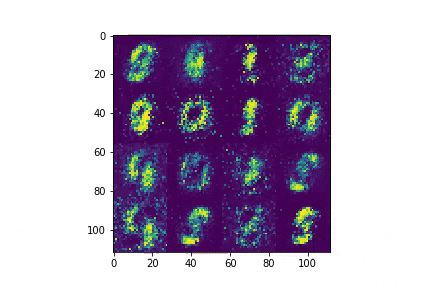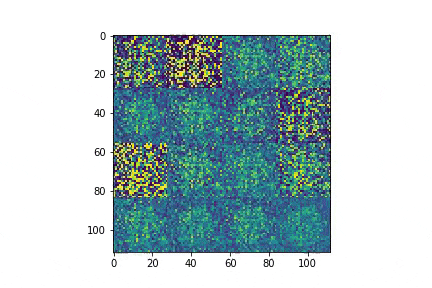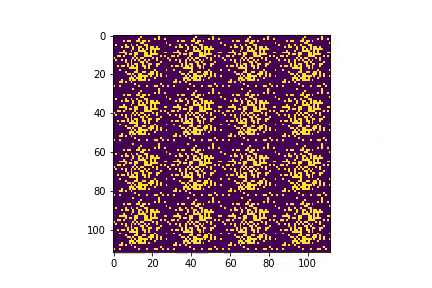# GANs 1/nI want to learn more about GANs. My experience with all things technical is that I learn fastest by trying to build stuff and then paying attention to what worked and what didn't. (For example: The single most positive change I ever made to my learning rate in math was to switch from  passively reading proofs in the book/paper to  actively trying to prove the result myself before reading on.) So I'm planning to implement different models / training tactics on simple data sets like MNIST. The advantage of a simple data set is that I can train models quickly, which tightens the feedback loop. Again, my experience is that tight feedback loops accelerate learning a lot.

I'm kicking things off with a plain vanilla, fully-connected GAN. This is similar in spirit to the OG gan paper. If you've done something like this before already, I think the most interesting part is at the end, where I look at the way that batch sizes and batch normalization affect the results.

## Training Setup

For this first implementation, I used fully connected networks for both the generator G and the discriminator D. G looks like this:

class G(nn.Module):

def __init__(self, in_dim=100, out_dim=28*28):
super().__init__()
self.model = nn.Sequential(
nn.Linear(in_dim, 512),
nn.LeakyReLU(0.2, inplace=True),
nn.BatchNorm1d(512),
nn.Linear(512, 512),
nn.LeakyReLU(0.2, inplace=True),
nn.BatchNorm1d(512),
nn.Linear(512, 512),
nn.LeakyReLU(0.2, inplace=True),
nn.BatchNorm1d(512),
nn.Linear(512, out_dim),
nn.Tanh()
)
self.in_dim = in_dim

def forward(self, z):
z = z.view(z.size(0), self.in_dim)
return self.model(z)

The network for D looks like this:

class D(nn.Module):

def __init__(self, in_dim=28*28, out_dim=1):
super().__init__()
self.model = nn.Sequential(
nn.Linear(in_dim, 512),
nn.LeakyReLU(0.2, inplace=True),
nn.Dropout(0.3),
nn.Linear(512, 512),
nn.LeakyReLU(0.2, inplace=True),
nn.Dropout(0.3),
nn.Linear(512, 512),
nn.LeakyReLU(0.2, inplace=True),
nn.Dropout(0.3),
nn.Linear(512, out_dim),
nn.Sigmoid()
)

def forward(self, x):
out = self.model(x.view(x.size(0), 28*28))
out = out.view(out.size(0), -1)
return out

The training loop is standard:

• Train D first, asking it to map real images from the data set to 1 and fake images from G to 0.
• Then train G, asking it to fool D into thinking that G's fake images are real.

I used the Adam optimizer for both G and D (default betas, learning rate of 2e-4), and optimized against BCELoss. I trained using a single Tesla V100 on GCP. Code here.

## Results

The resulting samples look reasonable to my eye:And here are the losses by epoch:## Things I Learned

### Batch Size Matters

There were a couple things that surprised me along the way. The first is that batch size matters more than I had thought. The images above came from a GAN trained with a batch size of 32. What if we increase the batch size to, say, 200? We'll leave all else equal.To my eye, these samples are worse and convergence takes longer. There's a stack exchange post on why this might happen. The argument is that larger batch sizes lead to "pointier" minima (in the sense that $$z = 100(x^2+y^2)$$ is pointier than $$z = x^2 + y^2$$), which generalize more poorly.

### Batch Norm Matters

What if we remove the batch norm layers from G so that it looks like this? (We've turned the batch size back to 32, btw.)

class G(nn.Module):

def __init__(self, in_dim=100, out_dim=28*28):
super().__init__()
self.model = nn.Sequential(
nn.Linear(in_dim, 512),
nn.LeakyReLU(0.2, inplace=True),
nn.Linear(512, 512),
nn.LeakyReLU(0.2, inplace=True),
nn.Linear(512, 512),
nn.LeakyReLU(0.2, inplace=True),
nn.Linear(512, out_dim),
nn.Tanh()
)
self.in_dim = in_dim

def forward(self, z):
z = z.view(z.size(0), self.in_dim)
return self.model(z)

We get mode collapse and poor performance from G:(I'm only showing 10 epochs here — typically, the changes to G(z) are minimal beyond this point. With more epochs, G's output stays the same, and it's loss gets worse and worse.)

### FC Layer Choices Matter Less

One thing that didn't matter much was the size of the FC layers. For example, the size of the FC layers in G above is

$\text{in_dim} \to 512 \to 512 \to 512 \to \text{out_dim}$

Changing this to, say

$\text{in_dim} \to 256 \to 512 \to 1024 \to \text{out_dim}$

didn't really change results. (For that experiment, I also changed D to

$\text{in_dim} \to 1024 \to 512 \to 256 \to \text{out_dim}$

since this is what I found most reference implementations did.) Of course, the layer sizes have to matter at some point, but the results were less sensitive to this than I had thought they'd be.